# x and y axis

x and y axis

The teacher drew a point on the blackboard and asked her students to identify it.

There were several responses from the students.Do you think these statements can fix the location of the point?

A point can be described in a horizontal way or a vertical way, which can be easily understood using a graph.

In this mini-lesson, we will learn about the $$x$$-axis and $$y$$-axis and what is x and y-axis geometry. Check out the interactive simulation to know more about the lesson and try your hand at solving a few interesting  interactivee questions at the end of the page.

## Lesson Plan

 1 X and Y-Axis Definition and Difference. 3 Thinking out of the Box! 4 Solved Examples on X and Y-Axis 2 Important Notes on X and Y-Axis 5 Interactive Questions on X and Y-Axis

## X and Y-Axis Definition and Difference

• X and Y-axis are the axes used in coordinate systems.
• They form a coordinate plane.
• The horizontal axis is represented by the x-axis and the vertical axis is represented by the y-axis.
• The point where the X and Y-axis intersect is known as the origin and is used as the reference point for the plane.
• The X-axis is also known as abscissa.
• The Y-axis is also known as ordinate.
• Any point on the coordinate plane is well defined by an ordered pair where the ordered pair is written as (x-coordinate,y-coordinate) or (x,y), where x-coordinate represents a point on the x-axis or perpendicular distance from the y-axis and y-coordinate represents a point on the y-axis or perpendicular distance from the x-axis.

X and Y-axis definition can be well understood by the following image: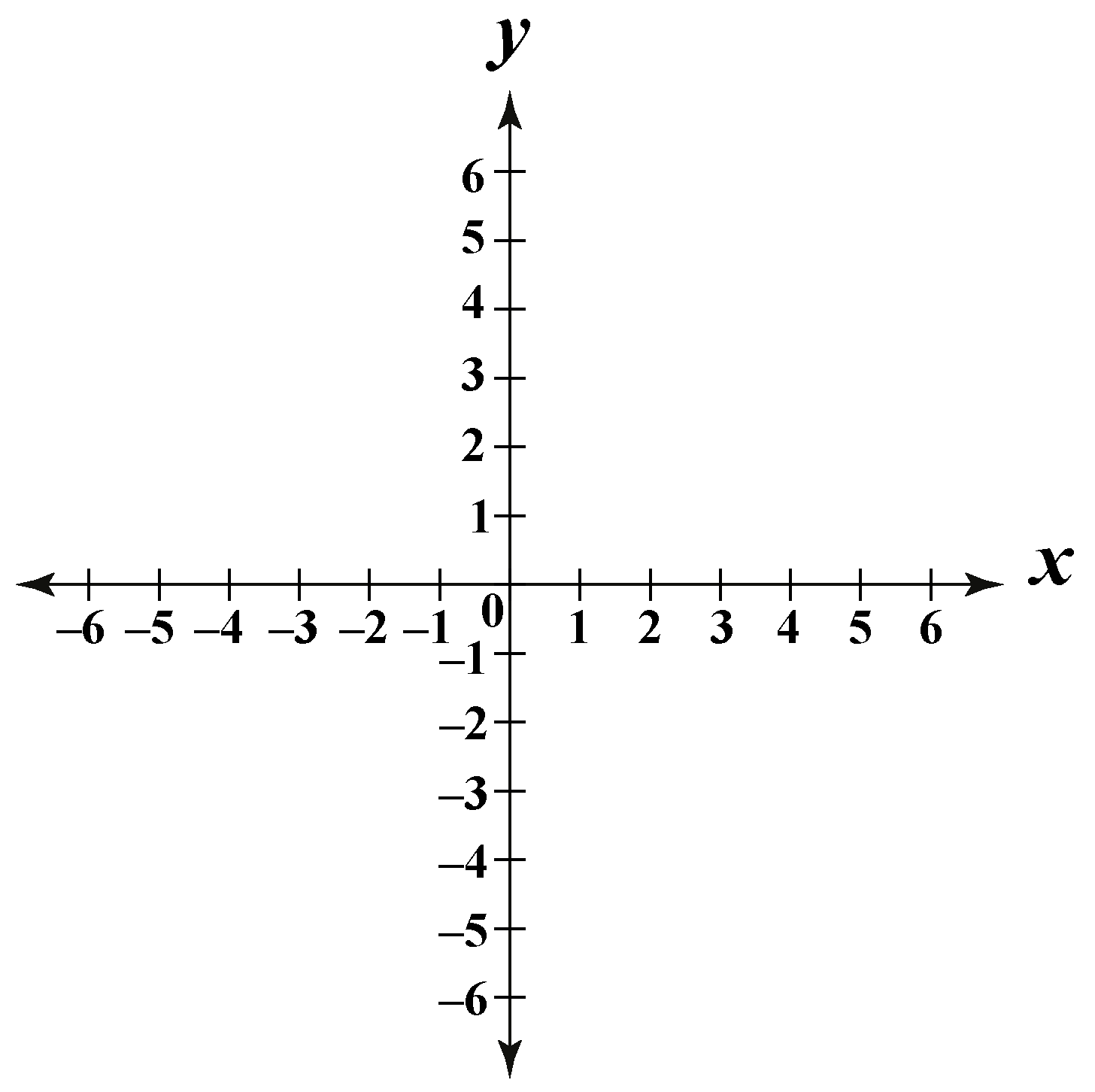This is also known as the x and y-axis graph.

Notable differences between the x and y-axis are given in the table below:

 X-Axis Y-Axis Known as abscissa Known as ordinate Referred to $$( \color{red}{x},y)$$ Referred to $$(x,\color{red}{y})$$

Examples

For example, the population of a city from 2015 to 2020 is given as:

 Years People in millions 2015 2016 2017 2018 2019 2020 1 1.5 2 2.5 3 3.5

Then to represent these points on the X and Y chart, using years on $$X$$-axis and the corresponding population on $$Y$$-axis as: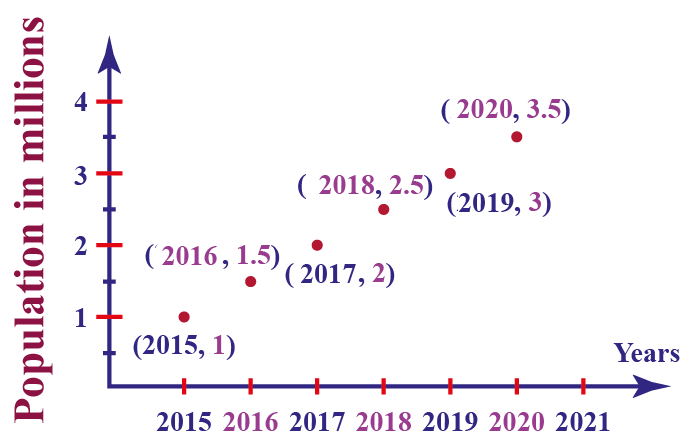## X and Y-Axis Graph Examples

Let's consider a linear equation $$y=2x+1$$.

Now to graph this equation construct a table having two columns for values of $$x$$ and $$y$$.

To draw the x and y-axis coordinate graph of the linear equation, we need to draw the X and Y-axis grid table for at least two points.

$$x$$ $$y$$
0 1
1 3
2 5

Now draw the points on the graph where the values of $$x$$ lie on $$X$$-axis and the corresponding values of $$y$$ lie on $$Y$$-axis.

Then join the points with a straight line to draw the graph of the equation.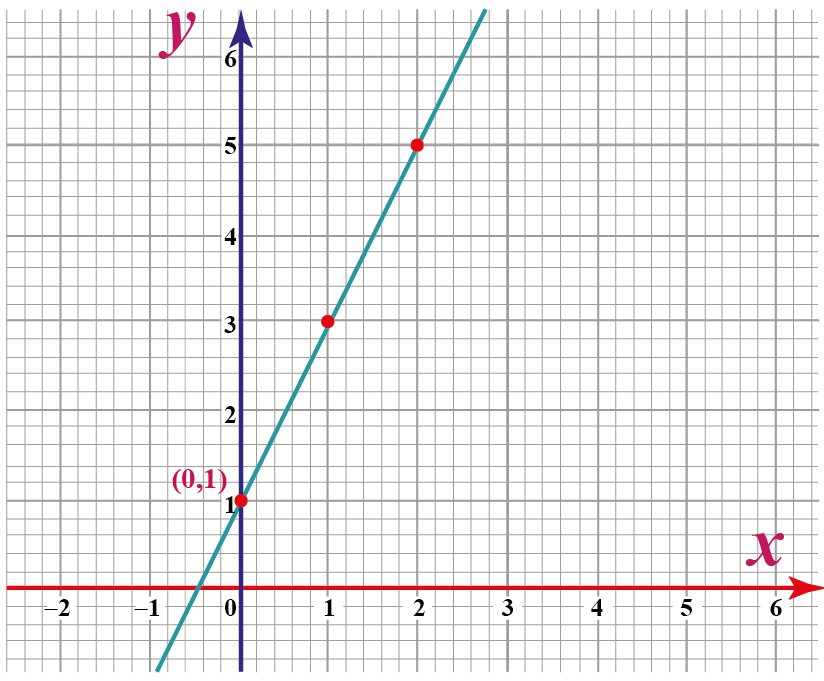Simulation

Let's try the simulation given below and see the various graphs of the linear equations.

To get the graph, input the two points and click on the draw button.

x and y axis

## What Comes First: X-Axis or Y-Axis?

To locate any point on the coordinate plane, we use an ordered pair where the ordered pair is written as (x-coordinate,y-coordinate) or (x,y), where x-coordinate represents a point on the x-axis or perpendicular distance from the y-axis and y-coordinate represents a point on the y-axis or perpendicular distance from the x-axis, therefore it is clear from above that x-axis comes first when writing the ordered pair to locate a point.

We can see here that the location of each point on the graph is noted as an ordered pair where the x-axis or x-coordinate leads the y-axis or y-coordinate.Think Tank
1. Can you predict the equation of $$x$$-axis?
2. Sam's age is 2 less than twice the age of his brother James. The age of James is $$x$$ years and the age of Sam is $$y$$ years.
Can you draw the graph of the equation of Sam's age on the X and Y graph chart?

## Solved Examples

 Example 1

Daniel is given an x and y-axis math problem by his teacher, where he has to plot the points $$(3,2)$$ and $$(2,3)$$ on a graph and draw a line passing through these points.

Can you determine the point where it meets $$x$$ axis?

Solution:

The points can be plotted on the graph as shown.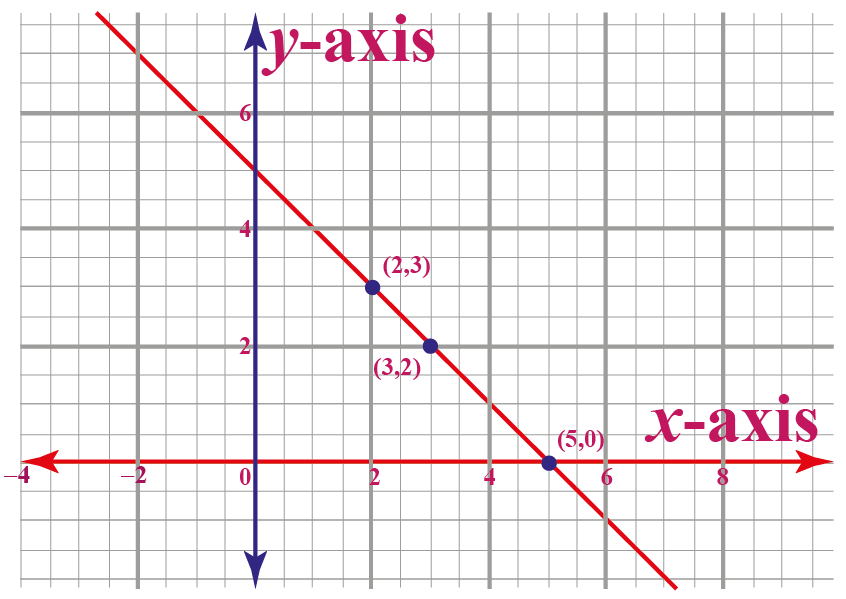The line meets the $$x$$-axis at the point (5,0).

 $$\therefore$$ The required point is (5,0).
 Example 2

Plot the points (0,2), (0,4.5), and (0,-3) on a coordinate system.

Do all the points lie on a line?

Can you name the line?

Solution:

The points on the graph are shown below.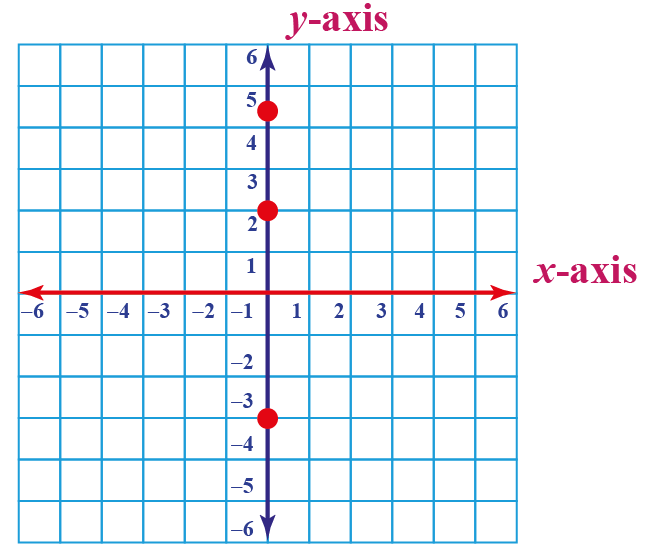Clearly, the points lie on a line.

All the points lie on $$y$$- axis.

 $$\therefore$$ The given points lie on $$y$$- axis.

## Interactive Questions

Here are a few activities for you to practice. Select/Type your answer and click the "Check Answer" button to see the result.

## Let's Summarize

The mini-lesson targeted the fascinating concept of the x and y-axis. The math journey around the x and y-axis starts with what a student already knows, and goes on to creatively crafting a fresh concept in the young minds. Done in a  way that is not only relatable and easy to grasp but will also stay with them forever. Here lies the magic with Cuemath. Now you will be able to easily solve problems on the x and y-axis quadrants and x and y-axis on a bar graph as well as x and y axis chart.

At Cuemath, our team of math experts is dedicated to making learning fun for our favorite readers, the students!

Through an interactive and engaging learning-teaching-learning approach, the teachers explore all angles of a topic.

Be it worksheets, online classes, doubt sessions, or any other form of relation, it’s the logical thinking and smart learning approach that we, at Cuemath, believe in.

### 1.  What are the 4 quadrants in a graph?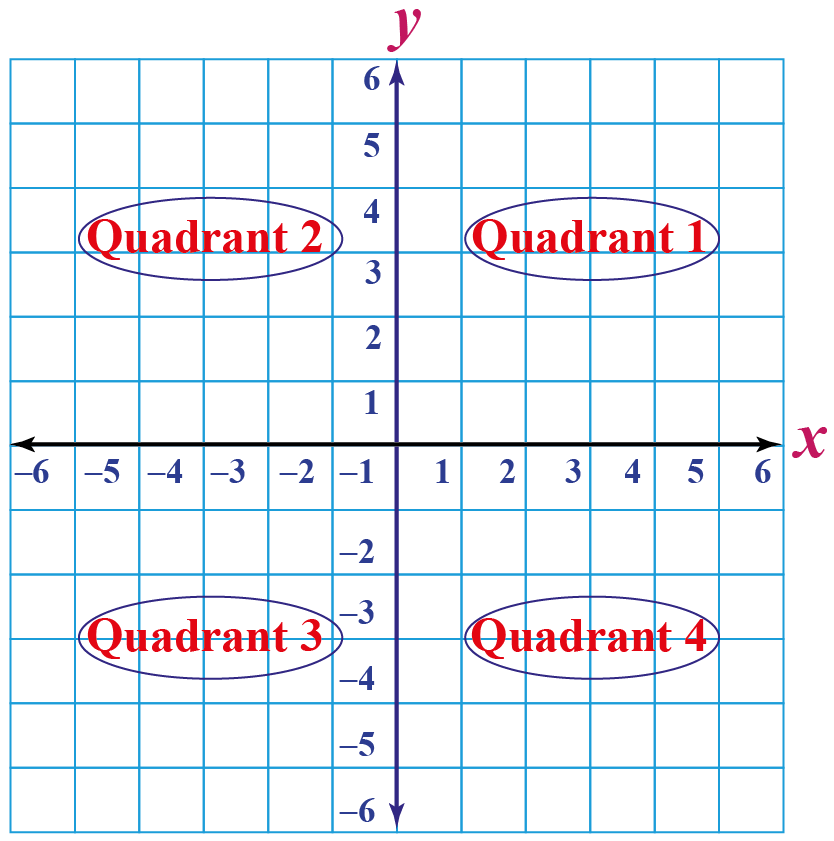### 2. How do I graph an equation?

To graph an equation, first construct a table having two columns for values of $$x$$ and $$y$$. Then draw the points on the graph where the values of $$x$$ lie on the x-axis and the corresponding values of $$y$$ lie on the y-axis. Then join the points to draw the graph of the equation.

### 3. Which point is on the negative $$y$$-axis?

The point which has a negative value of its $$y$$-coordinate is on the negative $$y$$-axis.

### 4. Which is x-axis and which is the y-axis?

The horizontal axis is known as the x-axis and the vertical axis is known as the y-axis.

### 5. How do you plot a graph with the x and y-axis?

At first, we draw and label the x and y-axis. Then we plot the coordinates of the function at various values of the x and y-coordinates. Then we connect the coordinates and plot the graph of a function.

### 6. What is the name of the y-axis?

The vertical axis is known as the abscissa-axis.

### 7. What is the name of the x-axis?

The x-axis is known as the ordinate-axis.

More Important Topics
Numbers
Algebra
Geometry
Measurement
Money
Data
Trigonometry
Calculus
More Important Topics
Numbers
Algebra
Geometry
Measurement
Money
Data
Trigonometry
Calculus